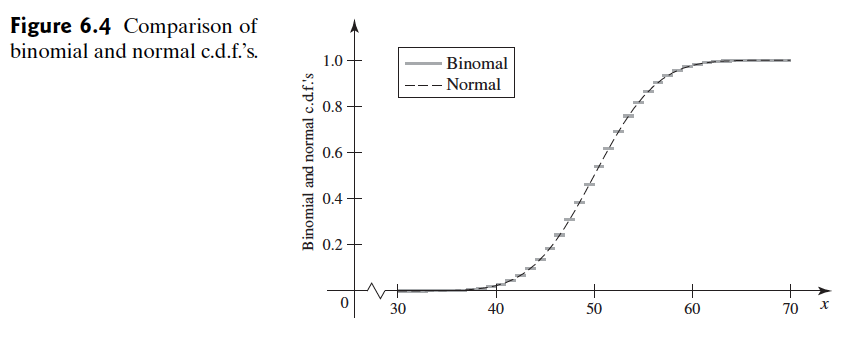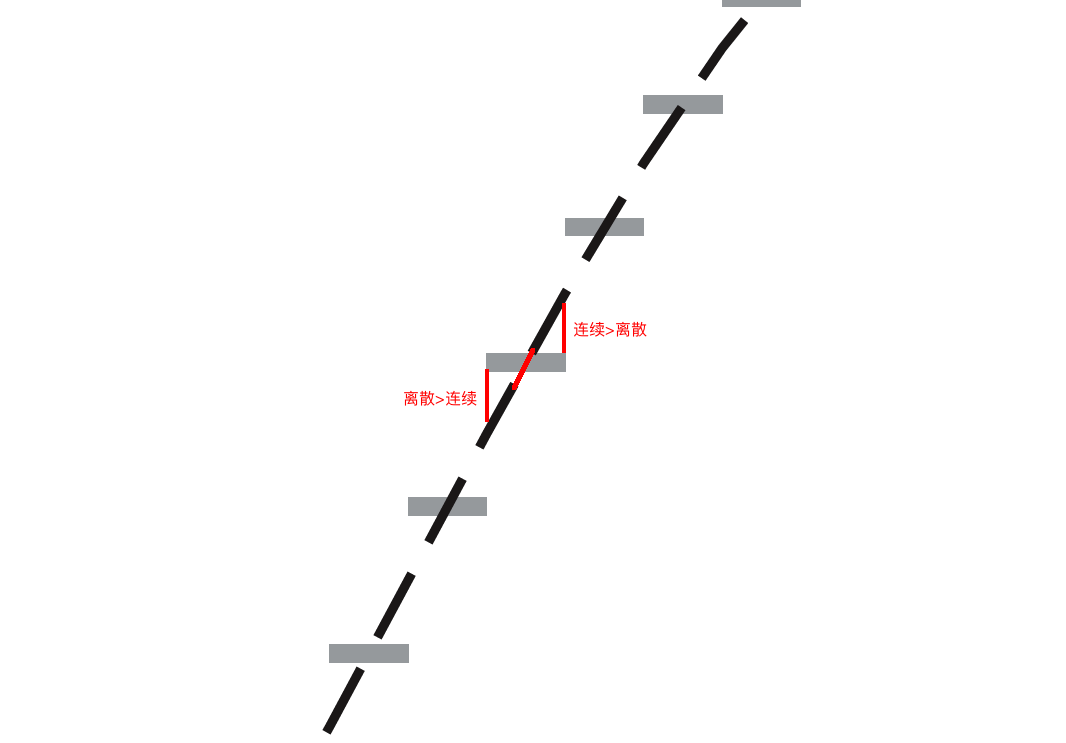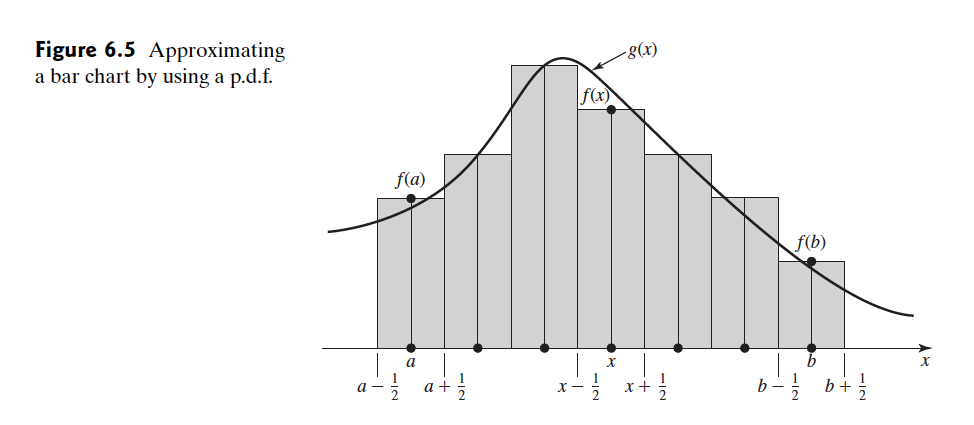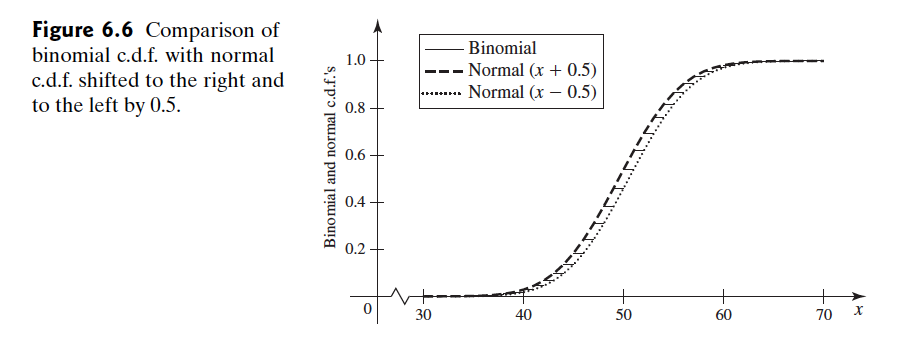Abstract: 本文介绍如何使用中心极限定理，将某区间上离散的随机变量，用一段连续的正态分布来近似
Keywords: The Central Limit Theorem，Approximation

# 分布连续性修正

## 连续分布近似离散分布 Approximating a Discrete Distribution by a Continuous Distribution

🌰 ：$$Pr(a\leq X\leq b)=\sum^{b}_{x=a}f(x)$$

$$Pr(a\leq Y\leq b)=\int^{b}_{a}g(x)dx\tag{6.4.2}$$

## 近似直方图 Approximating a Bar Chart$$Pr(a-\frac{1}{2} < Y < b+\frac{1}{2})=\int^{b+\frac{1}{2}}_{a-\frac{1}{2}}g(x)dx$$0%